# Content

## Electrical Circuits

### Resistive Circuits

#### Power and Energy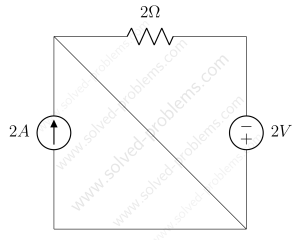Solving a Simple Circuit of Three Elements - A simple circuit is solved and power absorbed or supplied by each element is determined. KCL as well as Ohm's law are used in solving the circuit. positive sign convention is used in determining element powers. It is shown and discussed how a source, here current source, can be neither absorbing or supplying power. It is also mentioned that resistors are passive elements and always absorb power/energy.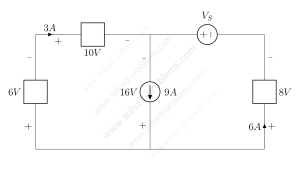Power and Energy Conservation - A circuit with two sources and three two port elements is studied. Some voltages and currents are given. Voltage of the voltage source is unknown. We need to analyze the circuit, find power of other elements and use the energy conservation to determine the power of the voltage source.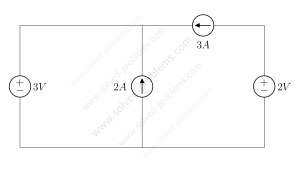Circuit Containing Only Sources - We go through solving a circuit which only containes independent sources: two voltage sources and two current sources. KVL and KCL are used to determine voltages and currents.

#### KVL / KCLSolve By Source Definitions, KCL and KVL - A DC resistive circuit consisting of one voltage source and one current source and three resistors is solved using source definition, KCL and KVL.Problem 1-12: Using Voltage Sources to Determine Node Voltages - A three-node circuit is solved by using the voltage of voltage sources. The nodal analysis is not used since there are enough voltage sources to determine all node voltages.

#### Thevenin's and Norton's Theorems/EquivalentFind Thevenin's and Norton's Equivalent Circuits - Thevenin's and Norton's equivalent circuits of a DC resistive circuit is determined. The circuit has an independent current source and a dependent voltage sThévenin's Theorem - Circuit with An Independent Source - Thevenin's Theorem is deployed to solve a quite simple circuit with only one independent voltage source. The solution is explained step-by-step.

#### Voltage/Current DivisionSolve Using Current Division Rule - A DC resistive circuit with two sources, one voltage source, one current source both independent solved using current division method.Voltage Divider - Voltage Division Rule - Voltage Division Rule: The voltage is divided between two series resistors in direct proportion to their resistance. This simple rule can be used in solving simple circuits or in simplifying the solution of complicated ones. In this post, the voltage divider circuit is studied and some examples are solved to show how this rule can be deployed in solving circuits.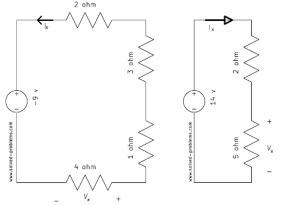Problem 1-16: Voltage Divider - In this solved problem, four circuits are solved using voltage divider (the voltage division rule). Problems are arranged from simple ones to more challenging ones. It is shown how voltage divider can be used to solve simple problems.

#### Circuit Reduction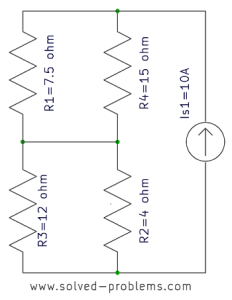Problem 1-9: Power of a Current Source - A simple DC resistive circuit is solved by circuit reduction (for series and parallel resistors) and the power a current source calculated.

#### SuperpositionFind the current using superposition. - Superposition rule is used to solve a DC resistive circuit which has two independent voltage sources and four resistors.Superposition method - Circuit with two sources - Finding current by using superposition method. There are two independent sources, so we need to turn them off one by one and calculate their contributions.Superposition Method - Circuit With Dependent Sources - A circuit with two independent and two dependent sources is solved by the superposition method. Independent sources are turned off one at a time and the contribution of the on source is calculated. Dependent sources should not be turned off.Superposition Problem with Four Voltage and Current Sources - A circuit with two voltage sources and two current sources is solved by the superposition method. The contribution of each source is calculated individually and the response is found by adding the contributions.

#### Mesh AnalysisMesh (Current) Analysis Problem - A circuit with four meshes solved using the mesh analysis. The circuit has two current sources, one voltage source and six resistors.Mesh Analysis - Supermesh - The mesh analysis used to solve the circuit which has a supermesh. After solving the circuit, power of sources determined.

#### Nodal Analysis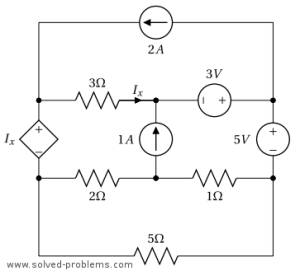Nodal Analysis - Circuit with Dependent Voltage Source - A 6-node circuit is solved with the nodal analysis. It contains one dependent voltage source, two independent voltage sources, two independent current sources and some resistors. The dependent causes two nodes to form a supernode.Nodal Analysis – 6-Node Circuit - A 6-node circuit is solved by the nodal analysis method and powers of voltage and current sources are calculated. The system of linear equations has three equations with three unknowns.Nodal Analysis - Dependent Voltage Source - A circuit with 3 nodes, one dependent voltage source, two current sources and three resistors is solved by the nodal analysis. The PSpice simulation result is also illustrated.Nodal Analysis - Dependent Current Source - Nodal analysis method is deployed to solve a four-node circuit which has a dependent current source. The target is to find the power of the controlled current source.Nodal Analysis - Dependent Voltage Source (5-Nodes) - The nodal analysis method is deployed to solve a circuit which has one dependent voltage source, one independent voltage source and one independent current source.Nodal Analysis - Supernode - This is a circuit with two voltage sources and two current sources. Nodal analysis method is used to solve the circuit. One of the voltage source forms a supernode between two nodes.Nodal Analysis Problem with Dependent Voltage and Current Sources - This is a complicated nodal analysis problem. The circuit has two independent sources and two dependent sources. A supernode is formed to solve the problem.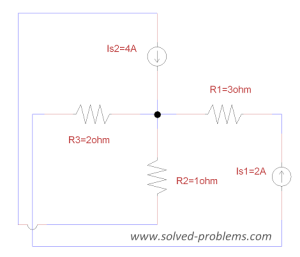Problem 1-8: Nodal Analysis - Power of Current Source - A simple DC resistive circuit with three resistors and two current sources are solved by the Nodal Analysis and power of one current source is determined.